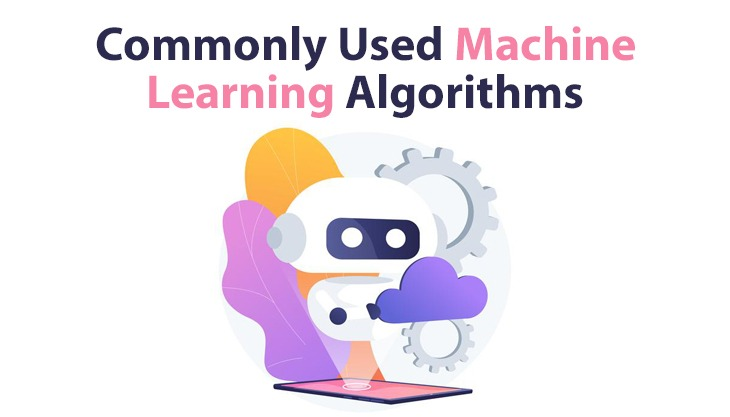# POSTS## Commonly Used Machine Learning Algorithms

Machine Learning is the study of complex computer and mathematical algorithms to automate analytical model building. It is considered a part of artificial intelligence. These machines can learn from the data, identify patterns and make decisions without being programmed explicitly and with minimum human interventions.

## WHAT ARE MACHINE LEARNING ALGORITHMS?

In machine learning, we use algorithms to turn a set of data into a model, which is then used to evaluate the unseen data or make predictions. The performance or accuracy of the model depends upon the type of the algorithms because different algorithms work differently according to the dataset and problem statements.

Machine Learning Algorithms are considered the core of machine learning. It is the algorithms that turn a dataset into a model, and the type of algorithm used, such as supervised, unsupervised, classification, regression, ensembling, boosting, etc. depends upon the problem statement you are working on, the type of data used for the problem, and, the computing resources available.

There are so many algorithms today; different algorithms can model a problem based on its interaction with the environment and the input data. We will go through them now:

There are three different types of machine learning algorithms based upon their learning styles, and they are:

1. Supervised Learning Algorithms

In Supervised Learning algorithms, we provide the input training data with answers, i.e., they have a known label or class such as spam/ not-spam, set of pictures of animals, and the animals' name stock price at a time.

The model is prepared through a training process whose goal is to correctly make predictions on data that it had not previously seen, such as identifying a picture of an animal included in the training dataset.

The model is trained until it achieves the desired level of accuracy on the training data and example of problems solved by supervised learning algorithms includes Regression, Classification, and Ensembling.

Example algorithms are Logistic Regression, Linear Regression, Random Forest, Support Vector Machines, etc.

2. Un-Supervised Learning Algorithms

In the Un-Supervised Learning Algorithm, the input data is not labelled, i.e., the algorithm goes through the information itself and does not have a known result. Still, they are used to solve more complex processing tasks compared to supervised learning algorithms.

The model is prepared by finding out patterns and hidden structures in the input data, for example, a set of clusters of data points, or it may be to organize data by similarity.

Example of problems solved by Un-supervised learning algorithms includes Clustering, Dimensionality Reduction, and Association Rule Learning.

Example algorithms are K-Means, PCA (Principal Component Analysis), Apriori Algorithm, etc.

3. Semi-Supervised Learning Algorithms

In Semi-Supervised Learning Algorithms, the training data combines a small amount of labelled data and many unlabelled data.

During the training process, the Semi-supervised learning algorithms learn to label unlabeled data points using the knowledge they have gained from a small number of labeled data points. The model should learn hidden patterns and must organize the data as well as the predictions.

Example of problems solved by Semi-Supervised Learning Algorithms includes Classification and Regression.

Example algorithms are mostly extensions to other flexible methods that predict the unlabeled data.

Note: In machine learning industry, you are most typically using supervised and unsupervised learning algorithms when working on most of the business problem statements, whereas semi supervised learning algorithms comes handy when you are working with computer vision and image processing tasks such as image classifications, and also with problem statements in which you have huge datasets with very few labeled examples.

### Use of Machine Learning Algorithms

Machine Learning algorithms are just like any other algorithms in computer science. ML algorithms are primarily described using maths and pseudocode in research papers and textbooks.

You can think of an algorithm as an optimization process that minimizes the model's error for the training dataset.

When new data is fed to these programs, they learn and optimize their performance by developing intelligence over time; They are used to analyze and process the input data to predict the output variables.

You will use multiple machine learning algorithms together in sporadic cases, and many ml practitioners use standard machine learning algorithms while working on their projects.

### Conclusion

In today's discussion, you discovered machine learning algorithms, types of machine learning algorithms, and the use of machine learning algorithms for solving your problem efficiently.